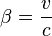## How to calculate speed of cosmic ray particles from kinetic energy

28 11 2011

In high energy and astroparticle physics energies for cosmic ray particles are given in GeV/n. But how much is that in term of speed?

First of all we must remember that these energies are kinetic energies (k).

The total energy E of a particle is the sum of its kinetic energy k and its mass m: E = m + k with c=1 and energy and mass measured with the same unit.

In special relativity: E = g m where g is the Lorentz factor that is equal to:andis the ratio between the speed of a particle and the speed of light c.

Remember that the mass of a proton is m=0.938 GeV. Lorentz factor is then given by:orand b is thenAssuming that mass is linear with number of nucleons in the nucleus, the same calculation applies to any ion using the kinetic energy per nucleon. We see that for energies bigger than 2 GeV/n particles travel almost at light speed (> 95 %).

 k g b 100 KeV 1.000107 0.0146 1 MeV 1.001066 0.04614 10 MeV 1.010661 0.14486 100 MeV 1.106610 0.42825 1 GeV 2.066098 0.87507 2 GeV 3.132196 0.94767 5 GeV 6.330490 0.98744 10 GeV 11.66098 0.99632 100 GeV 107.6098 0.99996This post was inspired by Protoni quasi veloci come la luce and Protoni quasi veloci come la luce: soluzione

Advertisements

### One response

4 04 2013

[…] Robert Millikan had already worked his way up the ranks when he picked up the assignment in the 1920′s.  Coining the term “cosmic rays,” Millikan believed gamma rays were the offender’s ammunition of choice.  But the ballistics didn’t check out.  In 1927 J. Clay dared to question the respected veteran by pointing out that cosmic rays were more intense at higher latitudes, swept there by the Earth’s magnetic field.  They couldn’t be light waves like gamma radiation; they had to be charged particles.  Experiments in the ’30′s, spurred by Bruno Rossi’s insights, showed that cosmic ray intensity was also greater from the west, indicating the particles were positively charged.  The weapon had been found.  High-speed protons.  But what could accelerate the tiny projectiles to such velocities, as high as 95% the speed of light? […]